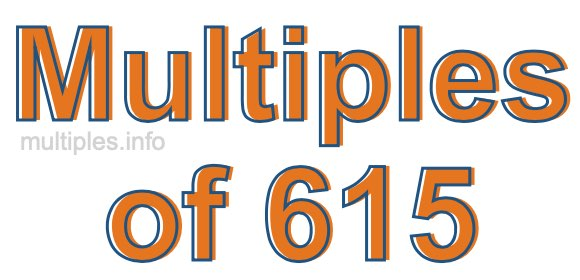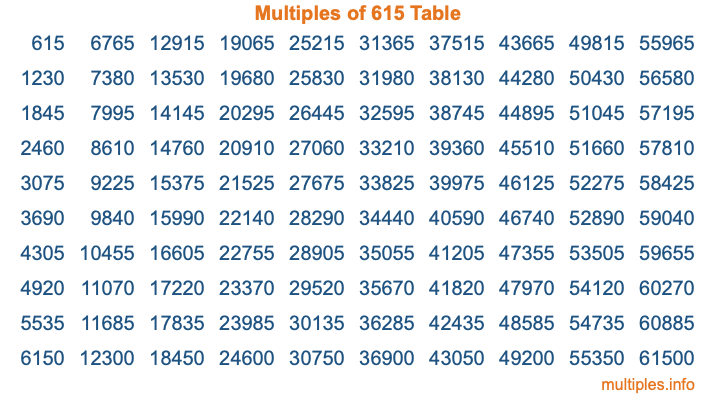Multiples of 615Welcome to the Multiples of 615 page. Here we will first teach you everything you will ever need to know about the multiples of 615, and then give you a study guide summary of everything we taught you to make sure you remember it all. Use this page to look up facts and learn information about the multiples of 615. This page will make you a multiples of six hundred fifteen expert!

Definition of Multiples of 615
Multiples of 615 are all the numbers that when divided by 615 equal an integer. Each of the multiples of 615 are called a multiple. A multiple of 615 is created by multiplying 615 by an integer.

Therefore, to create a list of multiples of 615, you start with 1 multiplied by 615, then 2 multiplied by 615, then 3 multiplied by 615, and so on for as long as you want. Thus, the list of the first five multiples of 615 is 615, 1230, 1845, 2460, and 3075. To see a larger list of multiples of 615, see the printable image of Multiples of 615 further down on this page. We also have a category where you can choose any nth multiple of 615.

Multiples of 615 Checker
The Multiples of 615 Checker below checks to see if any number of your choice is a multiple of 615. In other words, it checks to see if there is any number (integer) that when multiplied by 615 will equal your number. To do that, we divide your number by 615. If the the quotient is an integer, then your number is a multiple of 615.

Is  a multiple of 615?

Least Common Multiple of 615 and ...
A Least Common Multiple (LCM) is the lowest multiple that two or more numbers have in common. This is also called the smallest common multiple or lowest common multiple and is useful to know when you are adding our subtracting fractions. Enter one or more numbers below (615 is already entered) to find the LCM.

Check out our LCM Calculator if you need more details about the Least Common Multiple or if you need the LCM for different numbers for adding and subtraction fractions.

nth Multiple of 615
As we stated above, 615 is the first multiple of 615, 1230 is the second multiple of 615, 1845 is the third multiple of 615, and so on. Enter a number below to find the nth multiple of 615.

th multiple of 615

Multiples of 615 vs Factors of 615
615 is a multiple of 615 and a factor of 615, but that is where the similarities end. All postive multiples of 615 are 615 or greater than 615. All positive factors of 615 are 615 or less than 615.

Below is the beginning list of multiples of 615 and the factors of 615 so you can compare:

Multiples of 615: 615, 1230, 1845, 2460, 3075, etc.

Factors of 615: 1, 3, 5, 15, 41, 123, 205, 615

As you can see, the multiples of 615 are all the numbers that you can divide by 615 to get a whole number. The factors of 615, on the other hand, are all the whole numbers that you can multiply by another whole number to get 615.

It's also interesting to note that if a number (x) is a factor of 615, then 615 will also be a multiple of that number (x).

Multiples of 615 vs Divisors of 615
The divisors of 615 are all the integers that 615 can be divided by evenly. Below is a list of the divisors of 615.

Divisors of 615: 1, 3, 5, 15, 41, 123, 205, 615

The interesting thing to note here is that if you take any multiple of 615 and divide it by a divisor of 615, you will see that the quotient is an integer.

Multiples of 615 Table
Below is an image of the first 100 multiples of 615 in a table. The table is in chronological order, column by column. The first column has the first ten multiples of 615, the second column has the next ten multiples of 615, and so on.The Multiples of 615 Table is also referred to as the 615 Times Table or Times Table of 615. You are welcome to print out our table for your studies.

Negative Multiples of 615
Although not often discussed or needed in math, it is worth mentioning that you can make a list of negative multiples of 615 by multiplying 615 by -1, then by -2, then by -3, and so on, to get the following list of negative multiples of 615:

-615, -1230, -1845, -2460, -3075, etc.

Multiples of 615 Summary
Below is a summary of important Multiples of 615 facts that we have discussed on this page. To retain the knowledge on this page, we recommend that you read through the summary and explain to yourself or a study partner why they hold true.

There are an infinite number of multiples of 615.

A multiple of 615 divided by 615 will equal a whole number.

615 divided by a factor of 615 equals a divisor of 615.

The nth multiple of 615 is n times 615.

The largest factor of 615 is equal to the first positive multiple of 615.

615 is a multiple of every factor of 615.

615 is a multiple of 615.

A multiple of 615 divided by a divisor of 615 equals an integer.

615 divided by a divisor of 615 equals a factor of 615.

Any integer times 615 will equal a multiple of 615.

Multiples of a Number
Here you can get the multiples of another number, all with the same attention to detail as we did for multiples of 615 on this page.

Multiples of
Multiples of 616
Did you find our page about multiples of six hundred fifteen educational? Do you want more knowledge? Check out the multiples of the next number on our list!